## (10%) Problem 7: In a mass spectrometer, a specific velocity can be selected from a distribution by injecting charged particles between a se

Question

(10%) Problem 7: In a mass spectrometer, a specific velocity can be selected from a distribution by injecting charged particles between a set of plates with a constant electric field between them and a magnetic field across them (perpendicular to the direction of particle travel). If the fields are tuned exactly right, only particles of a specific velocity will pass through this region undeflected. Consider such a velocity selector in a mass spectrometer with a 0.095 T magnetic field.

What electric field strength, in volts per meter, is needed to select a speed of 4.1 x 10^6 ms?

in progress 0
6 months 2021-07-29T20:55:43+00:00 1 Answers 8 views 0

The strength of electric field is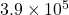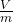Explanation:

Given:

Magnetic field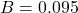T

Speed of particle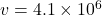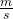Here we equate two forces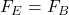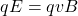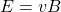For finding electric field strength,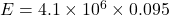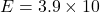Therefore, the strength of electric field is